Maths
Study Material

# Binomial Theorem

The Binomial Theorem is a technique (or method) of expanding a binomial expression that has been raised to some finite power.

6 minutes long
Posted by Kunduz Tutor, 27/10/2021Hesap Oluştur

Got stuck on homework? Get your step-by-step solutions from real tutors in minutes! 24/7. Unlimited.

The Binomial Theorem is a technique (or method) of expanding a binomial expression that has been raised to some finite power. Binomial theorem is a very crucial tool of expansion. Further, binomial theorem has its applications in algebraic expansion, algebra, permutation and combination, probability and even more.

Author – Ojasvi Chaplot

## Binomial Theorem Formula or Binomial Expansion

The representation of binomial theorem is as follows: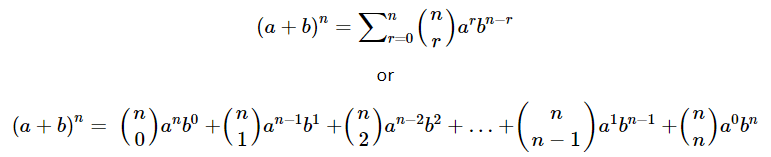Where,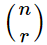or nCr or C(n, r) is Selection/Combination, i.e.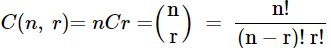Furthermore,

• n should be a positive integer.
•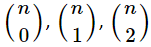,…or nC0nC1nC2,… are constants known as binomial coefficients.
• There are (n+1) number of terms in the expansion of (a+b)n
• The sum of powers of a and b in each individual term of the expansion (a+b)n is constant, i.e. it is equals to n.

For Example –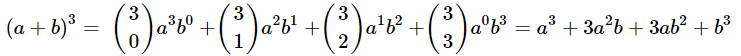### Binomial Expression

Any (algebraic) expression which contains two dissimilar (i.e. not similar) terms is termed as a binomial expression.

For Example –  (a+b)n, (a+b), etc.

## Binomial Theorem Coefficients

The binomial coefficients (or constants) in binomial theorem can also be obtained by the Pascal’s Triangle as –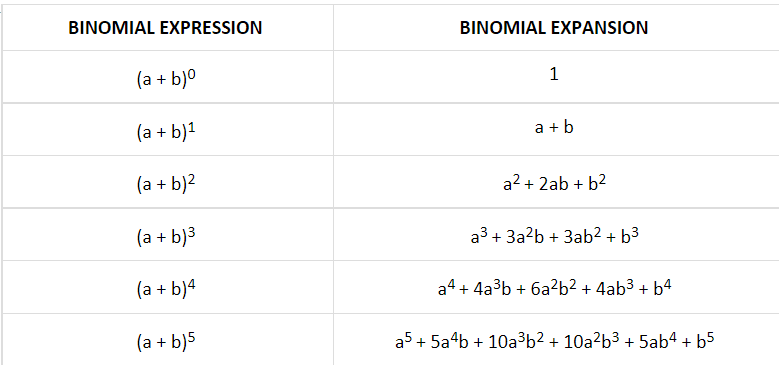And, likewise it goes on and on and on. Let’s take a look at the Pascal Triangle given below:

### Pascal Triangle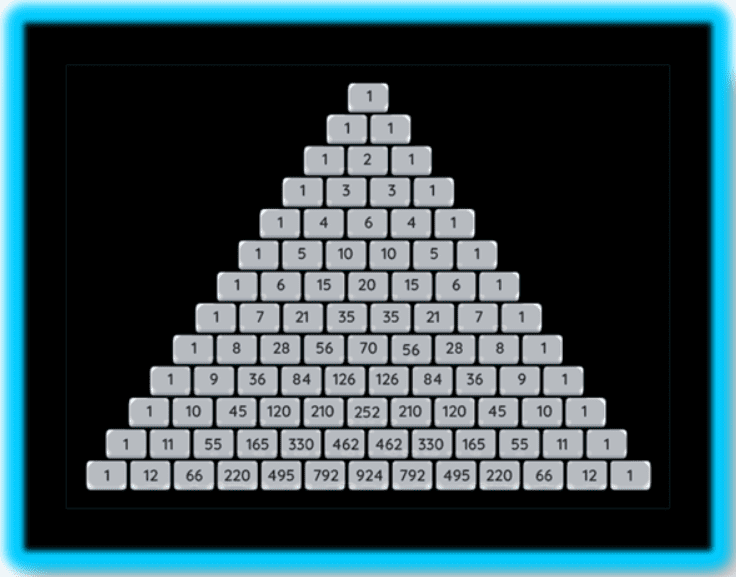### Properties of Binomial Coefficients

The binomial coefficients are the constant values (or numbers) associated with the variables a, b, in the expansion of (a + b)n in the binomial theorem. They are represented as nC0nC1nC2,….. The binomial coefficients are obtained through the pascal triangle or by using the combinations formula.

The properties of binomial theorem coefficients are as follows:

• nC0 = nCn = 1
• nCr = nCn-r
• nCx = nCy ; either x = y or x + y = n
• r × nCr = n × n-1Cr-1 (Very Important)
• nCr/ nCr-1 = (n – r + 1)/r
• nCr + nCr-1 n+1Cr

### Series of Binomial Coefficients in Binomial Theorem

The properties related to the series of binomial coefficients in binomial theorem are as follows:

• nC0 + nC1 + nC2 + nC3 + …+ nCn = 2n
• nC0 – nC1 + nC2 – nC3 + …+ nCn(–1)n = 0
• nC0 + nC2 + nC4 + nC6 + … = 2n-1
• nC1 + nC3 + nC5 + nC7 + … = 2n-1

Some more properties can also be obtained or can be used in calculation and simplification of binomial expansions in binomial theorems by using the generalized expansion of (a + b)n of binomial theorem and putting the values in place of a and b.

### Product of Binomial Coefficients in Series in Binomial Theorem

The properties related to the product of binomial coefficients in series in binomial theorem are as follows:

• nC02 + nC12 + nC22 + nC32 + …+ nCn2 = 2nCn
• nC0.nCn + nC1.nCn-1 + nC2.nCn-2 + …+ nCn.nC0 = 2nCn

This way, some more properties can also be obtained for the simplification of binomial expansions in binomial theorems by using the generalized expansion of (a + b)n of binomial theorem and substituting the values of a and b.

## Important Terms of Binomial Expansion

There are some important terminologies (or terms) related to the binomial expansion in the binomial theorem, which play a significant role in finding some of the specific terms in binomial theorem.

### General Term of Binomial Expansion in Binomial Theorem

The general term in binomial theorem of the expansion (a + b)n is denoted as –

Tr+1 = nCan-r br

### Independent Term of Binomial Expansion

To find out the term independent of a in the binomial expansion of (a + b)n, follow the given procedure:

• First of all, write down the general term as Tr+1 = nCan-r br
• Substitute the values of the given term in the general term and simplify it, then equate the exponent of a to 0, which will give us the correct answer.

### Middle Term(s) of Binomial Expansion

The middle term of the expansion (a + b)n in binomial theorem is obtained by following the given steps –

• CASE-I : If n is Even; => (n + 1) is odd.
• Then, T(n+1+1)/2 i.e. Tn/2 + 1 is the middle term of the binomial expansion.
• CASE-II : If n is Odd; => (n + 1) is even.
• Then, T(n+1)/2 and T[(n+1)/2 + 1] are the middle terms of the binomial expansion.

### Numerically Greatest Term/Coefficient of Binomial Expansion

The formula to find out the numerically greatest term in the expansion of (a + b)n is that first of all we find r, with the help of the formula –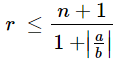; such that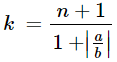and m = [k], where [.] denotes the greatest integer function.

• If k ∉ Z, then Tm+1 is numerically greatest term/coefficient.
• If k ∈ Z, then T, Tm+1 are numerically greatest terms/coefficients.

## Some Important Binomial Expansions

There are some important binomial expansions in the binomial theorem, which play a significant role in obtaining and simplifying specific expansions in binomial theorem.

• (1 + x)-1 = 1 – x + x2 - x3 + x4 - x5 + …….
• (1 – x)-1 = 1 + x + x2 + x3 + x4 + x5 + …….
• (1 + x)-2 = 1 – 2x + 3x2 - 4x3 +…….
• (1 – x)-2 = 1 + 2x + 3x2 + 4x3 +…….

## Picked For You

Furthermore, these are some topics which might interest you: